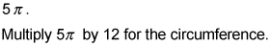##### SAT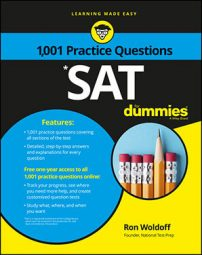The SAT Math exam may ask you to deal with, not a whole circle, but only part of it. For example, you may be asked to find the length of an arc (which is part of the circumference of a circle) or the area of a sector (which is part of the area of a circle).

The following practice questions ask you to find the area of a sector as a fraction of the circle, and the circumference of a circle based on the length of an arc.

## Practice questions

In the following figure, point O is the center of the circle.

1. What fraction of the circle is minor arc AOB?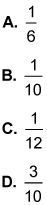2. If the length of minor arc AOB is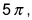what is the circumference of the circle?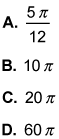## Answers and explanations

1. The correct answer is Choice (C). The entire circle is 360 degrees. Place the 30-degree angle over 360 degrees and reduce it to 1/12.
2. The correct answer is Choice (D). If minor arc AOB is 1/12 of the circle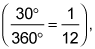then 1/12 of the circle is